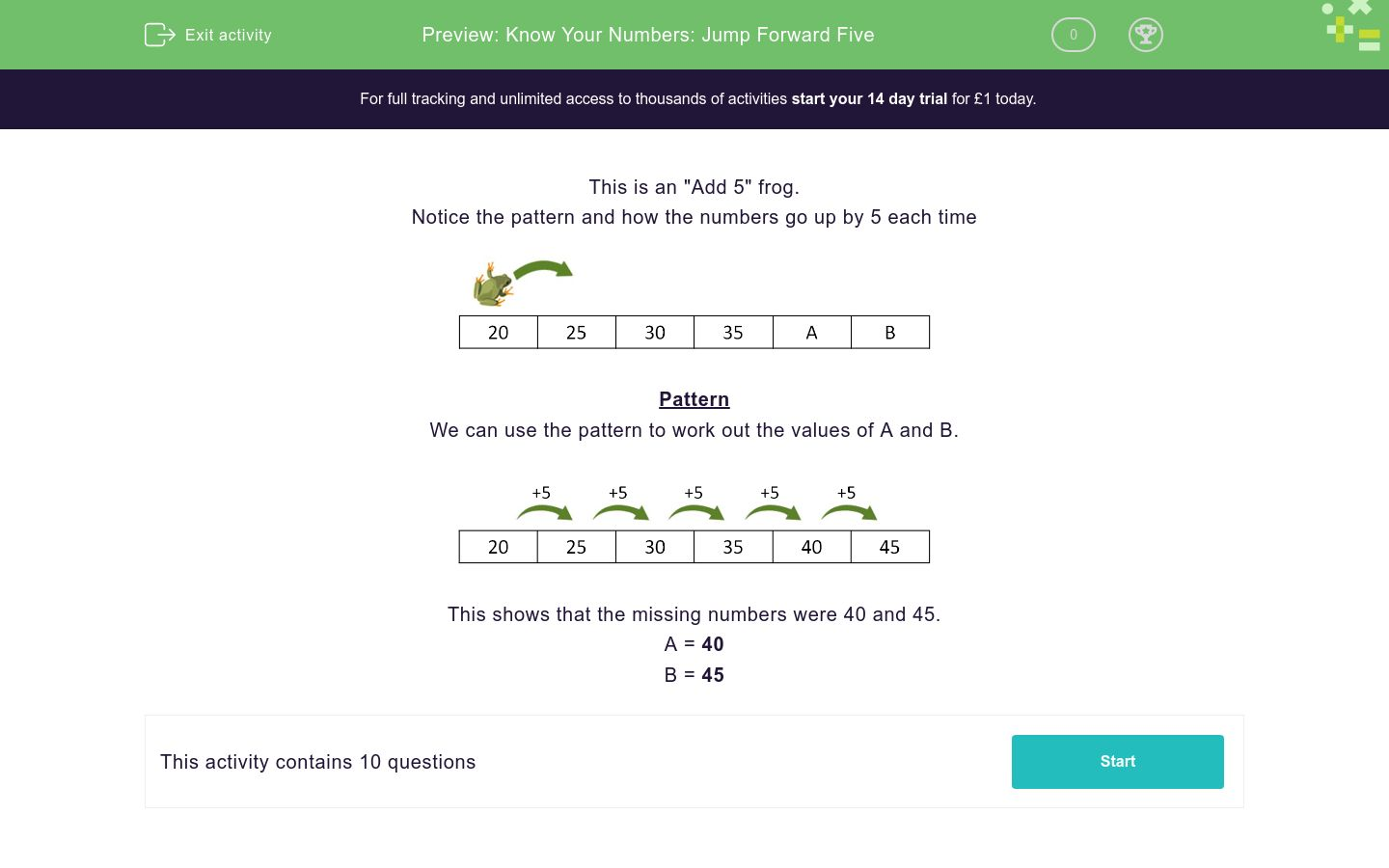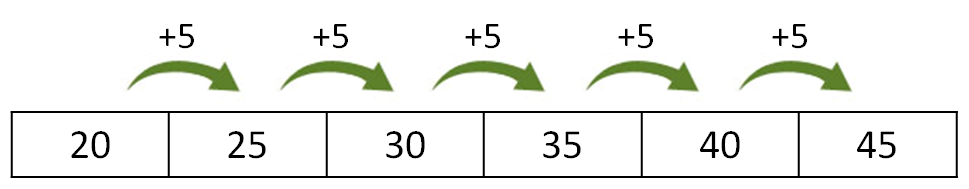# Know Your Numbers: Jump Forward Five

In this worksheet, students find the missing numbers in a sequence that goes up in fives.Key stage:  KS 1

Curriculum topic:   Number: Number and Place Value

Curriculum subtopic:   Count in Steps (2, 3, 5 and 10)

Difficulty level:### QUESTION 1 of 10

This is an "Add 5" frog.

Notice the pattern and how the numbers go up by 5 each timePattern

We can use the pattern to work out the values of A and B.This shows that the missing numbers were 40 and 45.

A = 40

B = 45

This is an "Add 5" frog.

Work out the values of A and B.A 17 B 27 32 37
 A B 12 23 22 11

This is an "Add 5" frog.

Work out the values of A and B.9 14 A B 29 34
 A B 19 22 24 12

This is an "Add 5" frog.

Work out the values of A and B.A 11 16 B 26 31
 A B 6 21 7 22

This is an "Add 5" frog.

Work out the values of A and B.12 17 A 27 B 37
 A B 21 22 32 33

This is an "Add 5" frog.

Work out the values of A and B.A 22 27 B 37 42
 A B 17 22 32 34

This is an "Add 5" frog.

Work out the values of A and B.3 A 13 B 28 33
 A B 9 18 8 17

This is an "Add 5" frog.

Work out the values of A and B.A B 28 33 38 43
 A B 17 22 18 23

This is an "Add 5" frog.

Work out the values of A and B.1 A 11 16 B 26
 A B 7 22 6 21

This is an "Add 5" frog.

Work out the values of A and B.A 12 17 B 27 32
 A B 7 8 23 22

This is an "Add 5" frog.

Work out the values of A and B.A 14 19 B 29 34
 A B 9 10 25 24
• Question 1

This is an "Add 5" frog.

Work out the values of A and B.A 17 B 27 32 37
 A B 12 23 22 11
• Question 2

This is an "Add 5" frog.

Work out the values of A and B.9 14 A B 29 34
 A B 19 22 24 12
• Question 3

This is an "Add 5" frog.

Work out the values of A and B.A 11 16 B 26 31
 A B 6 21 7 22
• Question 4

This is an "Add 5" frog.

Work out the values of A and B.12 17 A 27 B 37
 A B 21 22 32 33
• Question 5

This is an "Add 5" frog.

Work out the values of A and B.A 22 27 B 37 42
 A B 17 22 32 34
• Question 6

This is an "Add 5" frog.

Work out the values of A and B.3 A 13 B 28 33
 A B 9 18 8 17
• Question 7

This is an "Add 5" frog.

Work out the values of A and B.A B 28 33 38 43
 A B 17 22 18 23
• Question 8

This is an "Add 5" frog.

Work out the values of A and B.1 A 11 16 B 26
 A B 7 22 6 21
• Question 9

This is an "Add 5" frog.

Work out the values of A and B.A 12 17 B 27 32
 A B 7 8 23 22
• Question 10

This is an "Add 5" frog.

Work out the values of A and B.A 14 19 B 29 34
 A B 9 10 25 24
---- OR ----

Sign up for a £1 trial so you can track and measure your child's progress on this activity.

### What is EdPlace?

We're your National Curriculum aligned online education content provider helping each child succeed in English, maths and science from year 1 to GCSE. With an EdPlace account you’ll be able to track and measure progress, helping each child achieve their best. We build confidence and attainment by personalising each child’s learning at a level that suits them.

Get started